# Mathematically true (isTrue)

## Overview

The Mathematically true (isTrue) method checks whether an equation or inequality is true. The method is not a comparison method so no correct answer is set by the author. Instead when this method is used alone, any true equation or inequality entered by the learner will be marked as correct.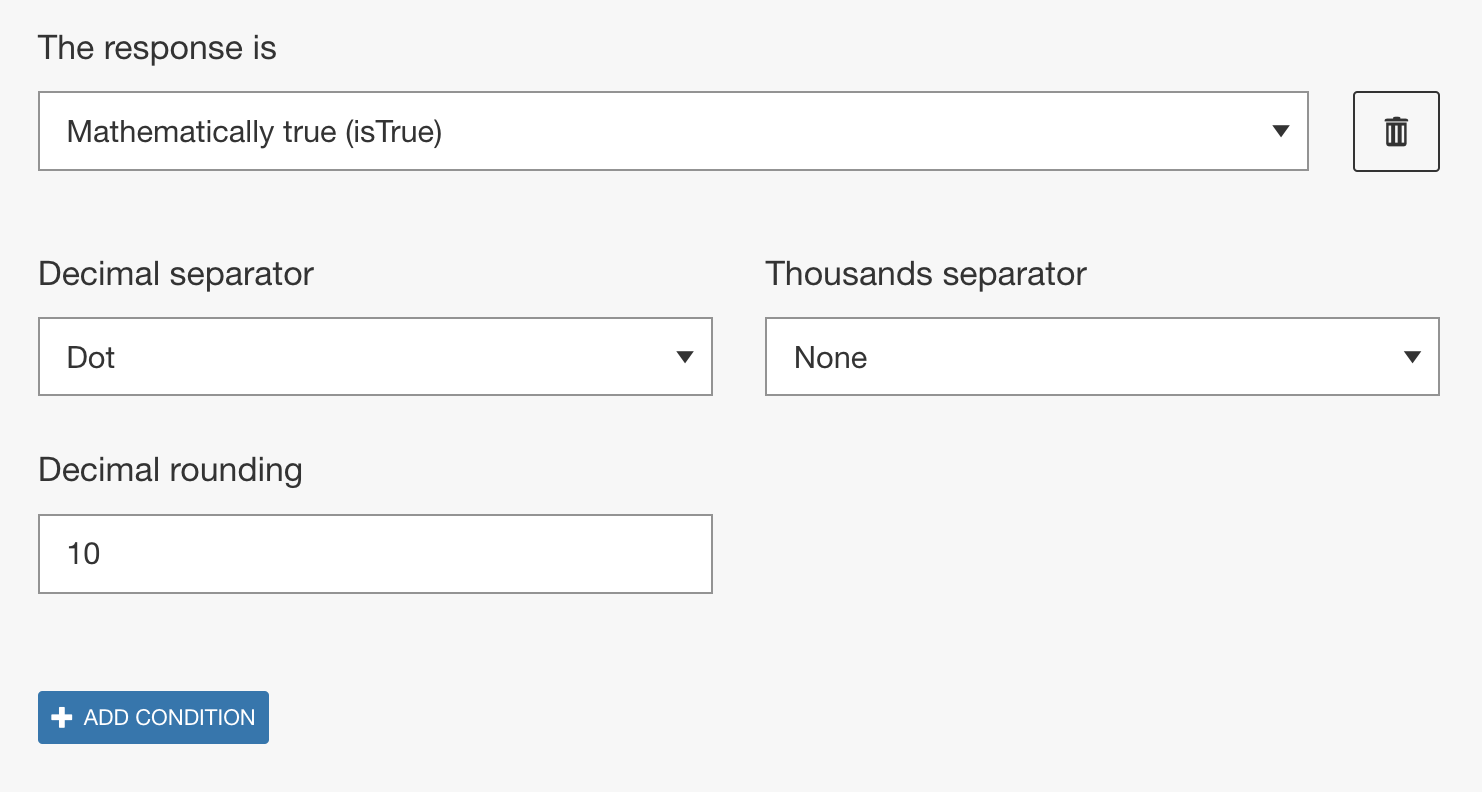Figure 1: The correct answer section for isTrue in Math Question type.

• Decimal and thousands separator
Authors can specify what separators should be recognized by the engine and can be used by the learner. From the Decimal separator drop-down either a dot or a comma can be selected. Depending on the selection of the decimal separator, from the Thousand Separator drop-down dot, comma, and/or space can be selected.
• Decimal rounding
This option specifies the number of decimal places the learner response is rounded to before evaluation. The maximum and default value is 10.

## Examples

#### Example 1

One way this method can be useful is if you've modified the response container to be an equation or inequality with response boxes for certain terms. Since the validation is set for the entire response container, the learner can enter any numbers that form a true equation. In this example we will ask the learner to provide two numbers that add to 10. In More options, we will set the Response container Formula template as follows: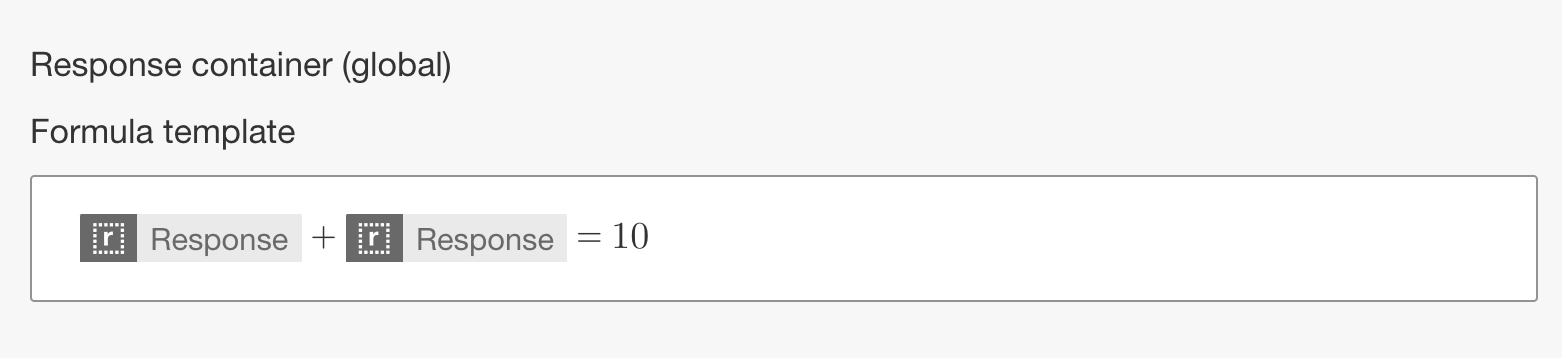Figure 2: Modifying the response container found in More options of Math question type.

As mentioned, any two numbers that add to 10 will be marked correct.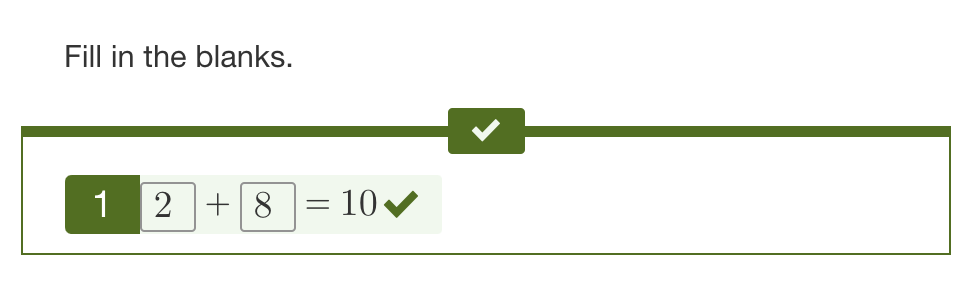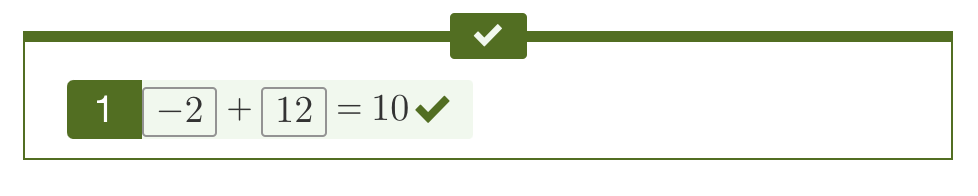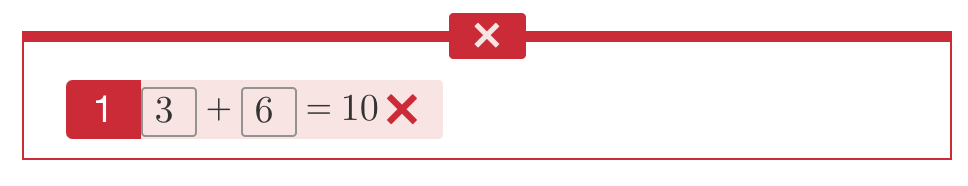Figure 3: Example learner responses and validation.